Home » Non-polyhedra

# Volume of Non-polyhedra

Non-polyhedra, are a category of geometric shapes which are formed when the solid has at least one curved surface or at least one surface that is not a polygon.

Below you can select the three-dimensional geometric figure of your interest, inside you will find a description, the volume formula of the 3D geometric solid and a volume calculator so you can calculate the volume of the solid automatically.

## Geometric shapes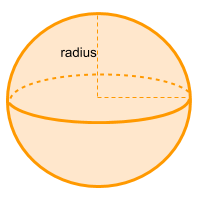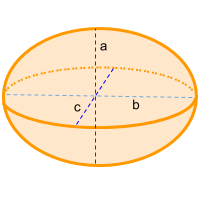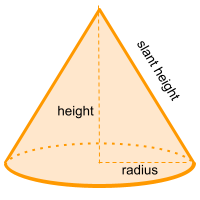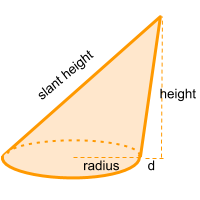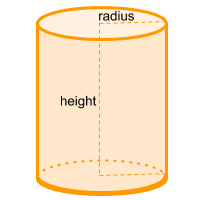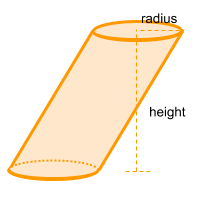Share